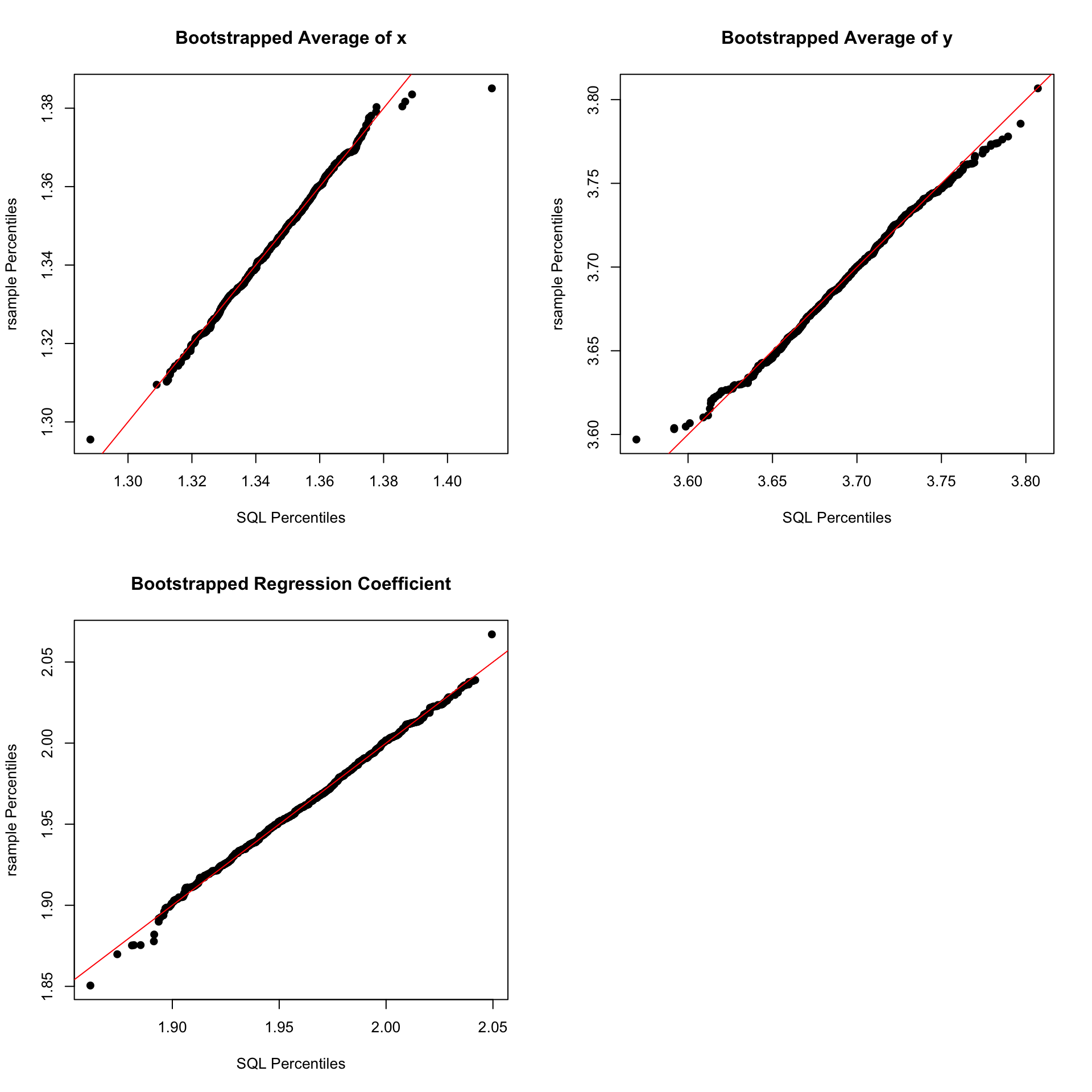# Bootstrapping in SQL

Statistics
Author

Demetri Pananos

Published

November 16, 2022

## Introduction

Remember the “Double Down” from KFC? It was bacon and cheese sandwiched between two deep fried pieces of chicken. I’m willing to bet we all conceived of it independently (as in “LOL wouldn’t it be crazy if…”), realistically could have made it ourselves, but were smart enough not to because “sure we could but… why?”.

This blog post is the Double Down of Statistics.

## Bootstrapping in SQL. No, Really.

Two things which have made my stats like easier:

• Bootstrapping, and
• Tidy data

R’s rsample::bootstraps seems to do one in terms of the other. Take a look at the output of that function. We have, in essence, one bootstrapped dataset per row.

library(tidyverse)
library(rsample )

rsample::bootstraps(cars)
# Bootstrap sampling
# A tibble: 25 × 2
splits          id
<list>          <chr>
1 <split [50/18]> Bootstrap01
2 <split [50/16]> Bootstrap02
3 <split [50/16]> Bootstrap03
4 <split [50/18]> Bootstrap04
5 <split [50/21]> Bootstrap05
6 <split [50/19]> Bootstrap06
7 <split [50/20]> Bootstrap07
8 <split [50/18]> Bootstrap08
9 <split [50/20]> Bootstrap09
10 <split [50/19]> Bootstrap10
# … with 15 more rows

In theory, I could unnest this and have one observation from each bootstrap per row, with id serving as an indicator to tell me to which resample the observation belongs to. Which means…I could theoretically bootstrap in SQL.

So, let’s do that. I’m going to use duckdb because its SQL-like and has some stats functions (whereas SQLite does not).

Let’s sample some pairs $$(x_i, y_i)$$ from the relationship $$y_i = 2x_i + 1 + \varepsilon_i$$, where the $$\varepsilon$$ are iid draws from a standard Gaussian Let’s stick that in a dataframe along with a row number column into our database. The data are shown in Table 1.

Table 1: My Data
original_rownum x y
1 2.09 5.73
2 0.97 3.58
3 0.70 1.37
4 1.44 4.84
5 2.07 4.14
6 1.67 3.09

To bootstrap in SQL, we need to emulate what the unnested results of rsample::bootstraps would look like. We need rows of (strap_id, original_data_rownum, and bootstrap_rownum). Let’s discuss the interpretation and purpose of each column.

• strap_id plays the part of id in rsample::bootstraps. We’re just going to group by this column and aggregate the resampled data later.

• original_data_rownum doesn’t really serve a purpose. It contains integers 1 through $$N$$ (where $$N$$ is our original sample size). We can do a cross join to get pairs (strap_id, original_data_rownum). This means there will be $$N$$ copies of strap_id, meaning we can get $$N$$ resamples of our data for each strap_id.

• bootstrap_rownum is a random integer between 1 and $$N$$. This column DOES serve a purpose, its basically the sampling with replacement bit for the bootstrap. Now, duckdb doesn’t have a function to sample random integers. To do this, I basically sample random numbers on the unit interval do some arithmetic to turn those into integers.

Let’s set that up now. The hardest part really is creating a sequence of numbers, but duckdb makes that pretty easy.

### Query To Make strap_id

-- Set up strap_ids in a table
CREATE OR REPLACE TABLE strap_ids(strap_id INTEGER);
-- Do 1000 bootstraps
INSERT INTO strap_ids(strap_id) select * from range(1, 1001, 1);
Table 2: Contents of strap_ids. These play the role of id in the rsample output.
strap_id
1
2
3
4
5

### Query To Make original_data_rownum

-- Set up original_data_rownum in a table
CREATE OR REPLACE TABLE original_data_rownum(original_rownum INTEGER);
-- I have 2500 observations in my data
INSERT INTO original_data_rownum(original_rownum) select * from range(1, 2500+1, 1);
Table 3: Contents of original_data_rownum. These play the role of id in the rsample output.
original_rownum
1
2
3
4
5

Ok, now we have the two tables strap_ids and original_data_rownum. All we need to do now is cross join then, and do the random number magic. That’s shown below in table Table 4.

### Query To Make bootstrap_rownum

create or replace table resample_template as
select
strap_ids.strap_id,
original_data_rownum.original_rownum,
-- I have 2500 observations in my data
round( -0.5 + 2501*random()) as bootstrap_rownum,
from
strap_ids
cross join
original_data_rownum;
Table 4: A sample from the table resampel_template.
strap_id original_rownum bootstrap_rownum
573 2216 4
312 2227 1792
2 554 1577
440 381 688
969 1352 1840

## Actually Doing The Bootstrapping: Its Just A Left Join!

Now all we have to do is join the original data onto resample_template. The join is going to happen on original_data.original_rownum = resample_template.bootstrap_rownum.

create or replace table resampled_data as
select
resample_template.strap_id,
resample_template.bootstrap_rownum,
original_data.x,
original_data.y
from
resample_template
left join
original_data on original_data.original_rownum = resample_template.bootstrap_rownum;

And congratulations, you have what is in essence an unnested rsample::bootstraps output. This happens shockingly fast in duckdb (actually, a bit faster than rsample does it, but that is anecdote I didn’t actually time them). The hard part now is the aggregation function. Obviously, you can’t do very complex statsitical aggregations in duckdb (or any other SQL dialect), but there are a few you can do. For example, let’s bootstrap the mean of $$x$$ and $$y$$, as well as the estimated regression coefficient.

select
'SQL' as method,
avg(x) as mean_x,
avg(y) as mean_y,
corr(y, x) * stddev(y) / stddev(x) as beta
from resampled_data
group by 1
order by 1;

We can easily compare the distributions obtained via the SQL bootstrap with distributions obtained from rsample::bootstrap

## But Does It Work

Yes…I think. The averages for $$x$$ and $$y$$ look really good, but the SQL bootstrap tails for the regression coefficient are a little thin.## Conclusion

This is pretty silly, and probably inefficient. I’m no data engineer, I’m just a guy with a Ph.D in stats and a lot of time on the weekend. I should get a hobby or something.